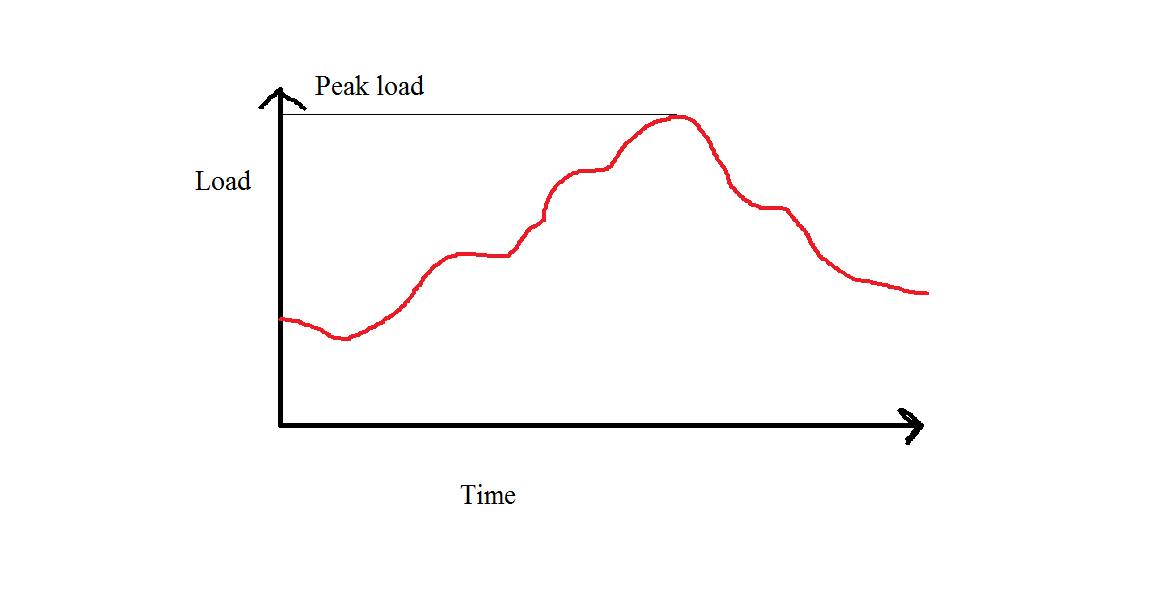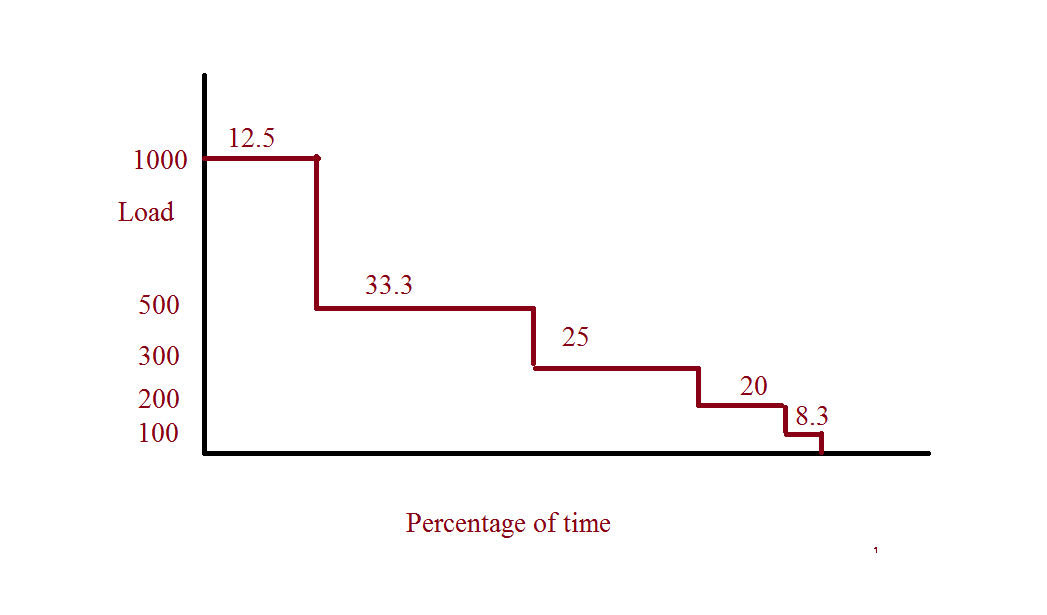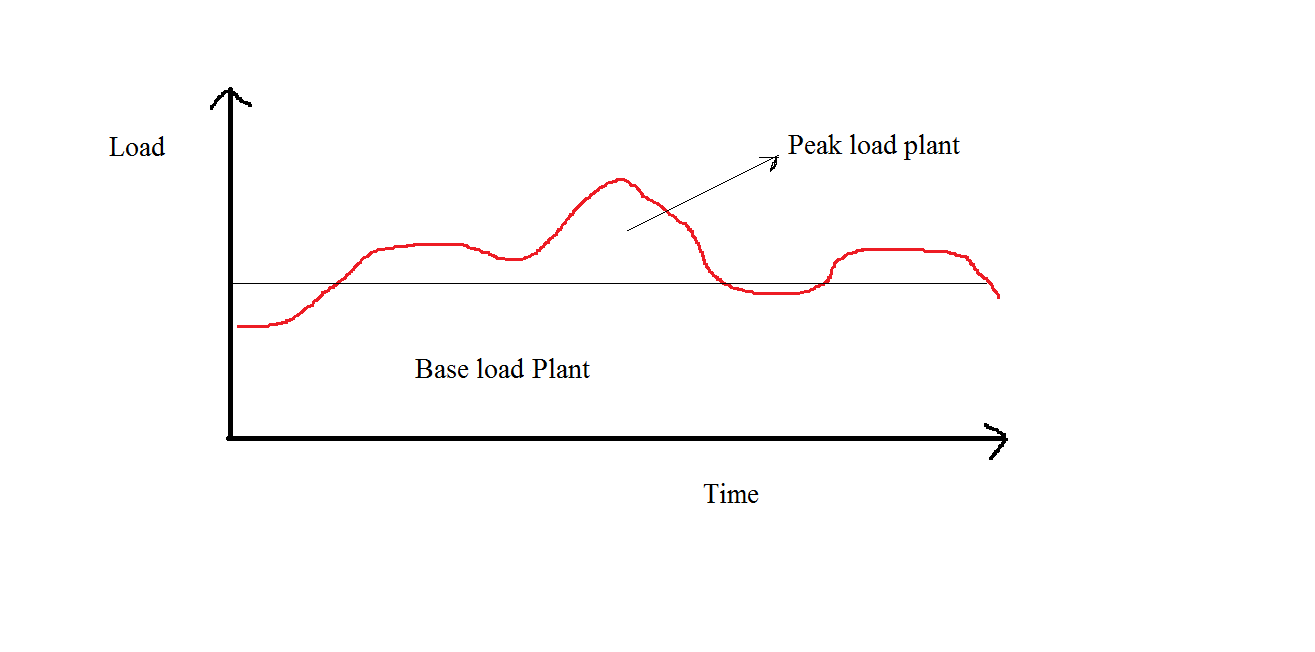## Economics of Electricity Generation

### Introduction

Economics is as important as technical knowledge for a electricity generation. Pricing the electricity generated is a truly complex issue. Because a lot of costs are involved in producing a unit of electricity from the coal in generation stations to transmission lines losses to distribution system losses and metering errors. Also the generation is not from a single source and each customer’s distance from the generating station varies so the losses he’s accountable for also vary. And these days owing to deregulation transmission distribution and generation are independent of each other. Thus finding a cost for unit of electricity is a  difficult job and an electrical engineer should always keep this in mind for at the end of day these prices should not be effected to develop any new technology.

Connected load is the load which is the total amount load which is connected to power supply. It is the sum of all rating of all loads connected in a home. When we apply for a new connection , we should mention the range/approximate connected load and thus utility or Discoms ( Distribution Companies ) maintain the connected load in area or under a substation.

2. ### Peak Demand :

It’s the amount of power demanded at a particular instant. This amount of demand is always available at all substation levels and also at generating stations. The peak demand ives an estimate how much power deficit we are in i.e. if the peak demand is not met at a particular instant or for a short duration , we are in deficit of power. In countries like India, this happens always. This means we do not have sufficient installed capacity to meet the maximum demand. This situation is meant by load shedding which means cutting of power supply to low priority loads ex : agriculture

3. Demand factor :  This is the ratio of the peak demand to the connected load. This can be less than or equal to one. But in real life scenarios as the connected load is not updated regularly it exceeds one.
4. Demand factor= Peak Demand/Connected load
5. Load curve : This the curve drawn by taking the demand at a particular instant on Y axis and the time on the X axis. This graph gives the energy usage pattern of the customers and many other factors which will used to plan the expansions. A typical load curve will look as follows

The time factor here creates some confusion hence generally for a dialy load cureve the demand is plotted for every 15 minutes and for yearly load curve the average day demand is taken for each day and plotted.### Diversity factor :

Here are some of the problems solved in the accompanying tutorial (at the end of the page):

Example 1 : A lighting installation has 15, 45 watts bulbs and operated as follows

• 5 bulbs  from 7pm -10 pm

• 6 bulbs from 10pm -11.30 pm

• 4 bulbs from 7pm -11.30pm

Determine the connected load, maximum demand and demand factor for the installation.

Also determine the change in the load factor if we use a 1 KW Air conditioner from 9pm -11pm.

The load curve gives us the idea of what load is during particular instant of in a day or over a year. It is plotted by taking the load at any time and for duration of time. Then a graph is plotted for the percentage of time of highest load and next less load on the next and least load at last of the curve. The curve obtained so is called load duration curve.

Problem 2  : Plot the load curve for following data

 Load 100 KW 500 Kw 1000Kw 200KW 300 kw Time 2hrs 8hrs 3hrs 5hrs 6hrs

Take the percentage for where 24hrs = 100%  and arrange in ascending order

 Load Hours Percentage 1000 3 12.5 500 8 33.33 300 6 25 200 5 20.8 100 2 8.33Generators :

1. Plant Capacity factor :

Plant Capacity factor= Actual Energy Generated Maximum capcity ×no of hours considered

1. Plant Use Factor :

(Solved in the tutorial at the end of the page)

Example : A power plant has two generators with capacities 1 MW and 2 MW each respectively. If the energy generated in a year is 300 lakhs units and the scheduled maintenance period  is 3 days , calculate PCF, PUF for the plant .

Assume the reserve capacity to be 500KWCost of generation

1. Fixed Cost

2. Running Cost

Fixed Cost : Fixed cost can also be sometimes called as capital cost because it is the cost involved to put the instrument in place here it is generator and other power plant equipment. To build a 500 MW plant it roughly takes 2500 crores. This amount is taken as capital cost to generate 500 MW of power.

Interest : The interest for the fixed cost also should be calculate and must be considered as a part of fixed cost.

Depreciation : Depreciation is another aspect to be considered as a part of fixed cost. When we buy a generator and use it for a long time, the efficincey falls due to degradation. The lower efficiency will result in high running costs and it will un economical to run the station. Also there may be new technologies coming up and other competitive plants will produce at higher efficiency .

To encounter all these, we take certain fund at the end of each year and accumulate it over this long period. This accumulated amount it generally used to replace the equipment and bring in new one which operate at higher efficiencies. Thus , we now conclude that a certain amount equal to the cost of generator should be accumulated over the life span of the generator’s life.

Let the initial cost of the equipment be C and final cost or scrap cost be S. Thus we need to accumulate the cost equivalent to (C-S).

Thus at the end of suppose P years, we need to get the amount of (C-S).

Now, we  need to consider the interest on this amount which is r %.

There are two methods in which this amount can be accumulated .

1. Straight line method

2. Sinking fund method

Straight line method : In this method, the cost to be accumulated in each year is constant. This means depreciation is uniform. Covered in detail in the tutorial (at the end of this page).

Sinking Fund Method : This is the second method to accumulate the depreciation over years. The disadvantage of the straight line method is that it is uniform throuought and the amount that needs to be paid initially is very high due to initial interest on all the capital cost and at the ending years, the depreciation is low. Thus this is against the fundamental physical depreciation which is low initially and very high at the end of life span. The sinking fund method offers to address this issue. Covered in detail in the tutorial provided (at the end of this page).

Running Cost : Running cost is the cost of the fuel which is used to generate the electricity and maintenance costs for the plan equipment and operation costs. The cost of the fuel is not always constant for a generator. It is not a linear function. Cost per  MW increases as the generation is increased. So the generators are run at best points where efficiency is high resulting in low cost of fuel. The operations costs include salaries and wages to the employees  and this cost practically remains constant for a generation. This is take under running costs because the employees are working for generation of electricity. Wages of clerical staff and management doesn’t come under running costs. Maintenance costs are the costs incurred in regular maintenance of machines. This includes cleaning of all water tubes in case of thermal power plants and generators bearings check and operations similar to this. Some of the staff and technical people are employed solely for maintenance and their salaries are also included.

Tariff for the customers

The fixed part depends on the maximum demand of the customer and determines the capacity of the plant Thus if there is higher maximum demand the fixed cost will increase and if the maximum demand is not maintained throughout the running costs alos increase because of load utilization factor is low which results in low plant utilization factor. Another important consideration is Power Factor. If the loads are operated at low power factor, then higher current is required to supply same amount of KW. Thus increasing transmission losses. Hence there is a separate tariff in some places in place to include the effect of power factor. In Cases like India, People are advised to use synchronous capacitors to improve the power factor at the consumer end.

Cost to customer  = A Rs/KW + B Rs/KWH

Solution provided in the tutorial provided (at the end of this page).

Example : A plant costs  Rs 80,000 and has a useful life of 15 years. If the salvage value is 5,000 determine the amount  which should be saved annually for both methods : sinking fund and straight line. Assume 5% rate of interest.

Solution provided in the tutorial provided (at the end of this page).

Problem :

1. Fuel :5 * 10^6

2. Generation = 2.4 * 10^6

3. Transmission  = 5 * 10^6

4. Distribution = 3 * 10^6

Of these items 90% 10% 5% and 7% respectively are allocated to running costs the remainder being a fixed cost. Total loass between station and consumers is 10 % . If load factor of station is raised to 40% for same maximu demand find the saving in cost per Kwhr

Solution provided in the tutorial provided (at the end of this page).

Problem :

Solution provided in the tutorial provided (at the end of this page).

Problem : An I industrial organization requires  5 ×106   Kwh per month with a maximum demand of 1MW. Determine the annual cost of energy

1. If supplied by govt utility at a rate of 100 per KW of maximum demand  plus 4 paisa per unit

2. If supplied from a private entity at rs 600per KWand running cost of 7 paisa per unit. Interest and depreciation is 18 %. Also calculate overall cost .

Complete Tutorial with Problems and Solutions :